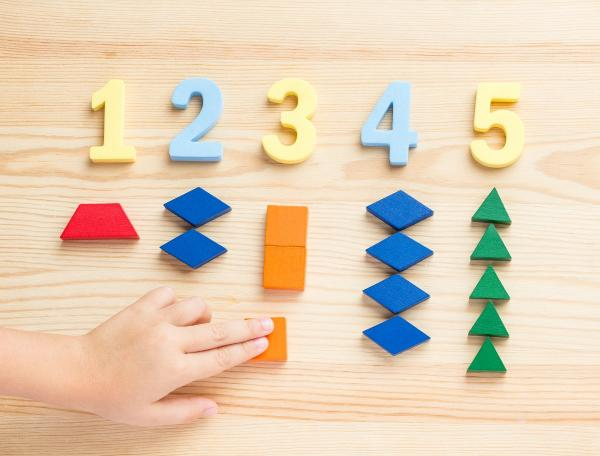# A maths toolkit

6 - 8 yearsDeveloped in partnership with Education Services Australia

The Australian Curriculum sets the goal for what all students should learn as they progress through their school life. Skills in the Year 1-2 curriculum include:

• estimating the solution to a problem with one- and two-digit numbers, and then calculating the answer
• using materials to model addition and subtraction problems involving one- and two-digit numbers
• recalling combinations of two numbers that add up to 10, and using those combinations to work out combinations up to 20
• modelling simple division and multiplication problems by making equal groups with materials, or arranging items in equal rows and columns (arrays)
• counting by 2s, 5s, 10s, and later 3s.

It’s easy to help your child practise these skills as part of everyday life – just use these simple ideas.

## Develop their toolkit

Your budding mathematician is developing a toolkit of strategies and shortcuts for solving maths problems. To help them learn what tool to use when, talk about how you are solving maths problems, and ask them to help you. Try to include practice at:

• estimating an answer first, then working it out and comparing the two answers
We need five ribbons that are 17cm long each. 17 is a bit less than 20, and 5 times 20 is 100, so 1 metre of ribbon should be enough. Do you want to check on the calculator?
• using materials to model the problem
Hmm, we need to work out ‘14 minus 8’. Let's put out 14 sultanas, eat 8, and count how many are left!
• practising the combinations of two numbers that add up to 10 (1+9, 2+8, 3+7, 4+6, 5+5) and using those shortcuts to work out combinations up to 20
• counting by 2s, 5s, 10s and (later) by 3s
• finding different ways to work out how many units there are in a group
Let's see, how many ways there are to work out how many eggs are in the egg carton? That's right, you can count by ones, or you can count by twos, or you can add together the number in each row. Which way do you like best?

## Go online

For online reinforcement, Can you add up to 10? will give your child practice at:

• exploring number combinations up to 10 through stories and songs.

The difference bar will give your child practice at:

• exploring ways to compare, add and subtract two-digit numbers in their head.

[1-2Learning]

Toolkits
Tags